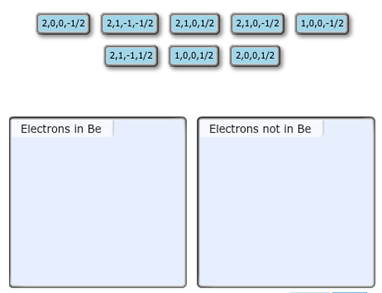Problem: Each set is ordered(n,l,ml,ms). Identify the sets of quantum numbers that describe all the electrons in the ground state of a neutral beryllium atom, Be. Each set is ordered(n,l,ml,ms). Choose the appropriate items to their respective bins.

FREE Expert Solution
98% (447 ratings)
Problem Details

Each set is ordered(n,l,ml,ms). Identify the sets of quantum numbers that describe all the electrons in the ground state of a neutral beryllium atom, Be. Each set is ordered(n,l,ml,ms). Choose the appropriate items to their respective bins.What scientific concept do you need to know in order to solve this problem?

Our tutors have indicated that to solve this problem you will need to apply the The Electron Configuration: Quantum Numbers concept. You can view video lessons to learn The Electron Configuration: Quantum Numbers Or if you need more The Electron Configuration: Quantum Numbers practice, you can also practice The Electron Configuration: Quantum Numbers practice problems .

What is the difficulty of this problem?

Our tutors rated the difficulty of Each set is ordered(n,l,ml,ms). Identify the sets of quantum... as high difficulty.

How long does this problem take to solve?

Our expert Chemistry tutor, Dasha took 5 minutes to solve this problem. You can follow their steps in the video explanation above.

What professor is this problem relevant for?

Based on our data, we think this problem is relevant for Professor Jones' class at University of Guelph.